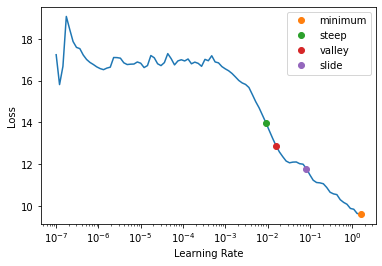# Hyperparam schedule

Callback and helper functions to schedule any hyper-parameter
``from fastai.test_utils import *``

## Annealing

source

### annealer

`` annealer (f)``

Decorator to make `f` return itself partially applied.

This is the decorator we will use for all of our scheduling functions, as it transforms a function taking `(start, end, pos)` to something taking `(start, end)` and return a function depending of `pos`.

source

### sched_exp

`` sched_exp (start, end, pos)``

source

### sched_no

`` sched_no (start, end, pos)``

source

### sched_cos

`` sched_cos (start, end, pos)``

source

### sched_lin

`` sched_lin (start, end, pos)``
``````annealings = "NO LINEAR COS EXP".split()
p = torch.linspace(0.,1,100)
fns = [SchedNo, SchedLin, SchedCos, SchedExp]``````
``````for fn, t in zip(fns, annealings):
plt.plot(p, [fn(2, 1e-2)(o) for o in p], label=t)
f = SchedPoly(2,1e-2,0.5)
plt.plot(p, [f(o) for o in p], label="POLY(0.5)")
plt.legend();``````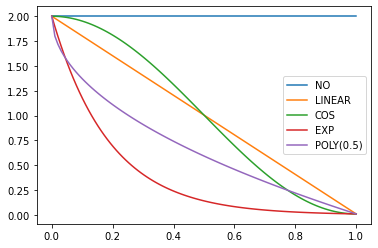source

### SchedLin

`` SchedLin (start, end)``

Linear schedule function from `start` to `end`

``````sched = SchedLin(0, 2)
test_eq(L(map(sched, [0., 0.25, 0.5, 0.75, 1.])), [0., 0.5, 1., 1.5, 2.])``````

source

### SchedCos

`` SchedCos (start, end)``

Cosine schedule function from `start` to `end`

``````sched = SchedCos(0, 2)
test_close(L(map(sched, [0., 0.25, 0.5, 0.75, 1.])), [0., 0.29289, 1., 1.70711, 2.])``````

source

### SchedNo

`` SchedNo (start, end)``

Constant schedule function with `start` value

``````sched = SchedNo(0, 2)
test_close(L(map(sched, [0., 0.25, 0.5, 0.75, 1.])), [0., 0., 0., 0., 0.])``````

source

### SchedExp

`` SchedExp (start, end)``

Exponential schedule function from `start` to `end`

``````sched = SchedExp(1, 2)
test_close(L(map(sched, [0., 0.25, 0.5, 0.75, 1.])), [1., 1.18921, 1.41421, 1.68179, 2.])``````

source

### SchedPoly

`` SchedPoly (start, end, power)``

Polynomial schedule (of `power`) function from `start` to `end`

``````sched = SchedPoly(0, 2, 2)
test_close(L(map(sched, [0., 0.25, 0.5, 0.75, 1.])), [0., 0.125, 0.5, 1.125, 2.])``````
``````p = torch.linspace(0.,1,100)

pows = [0.5,1.,2.]
for e in pows:
f = SchedPoly(2, 0, e)
plt.plot(p, [f(o) for o in p], label=f'power {e}')
plt.legend();``````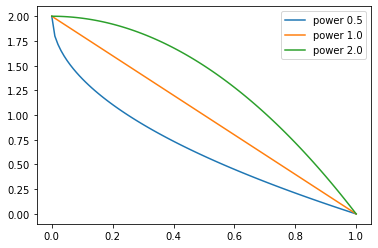source

### combine_scheds

`` combine_scheds (pcts, scheds)``

Combine `scheds` according to `pcts` in one function

`pcts` must be a list of positive numbers that add up to 1 and is the same length as `scheds`. The generated function will use `scheds` from 0 to `pcts` then `scheds` from `pcts` to `pcts+pcts` and so forth.

``````p = torch.linspace(0.,1,100)
f = combine_scheds([0.3,0.7], [SchedCos(0.3,0.6), SchedCos(0.6,0.2)])
plt.plot(p, [f(o) for o in p]);``````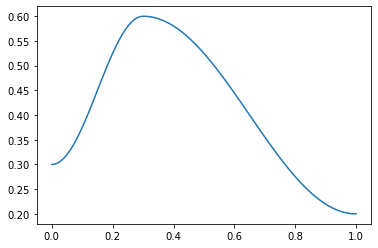``````p = torch.linspace(0.,1,100)
f = combine_scheds([0.3,0.2,0.5], [SchedLin(0.,1.), SchedNo(1.,1.), SchedCos(1., 0.)])
plt.plot(p, [f(o) for o in p]);``````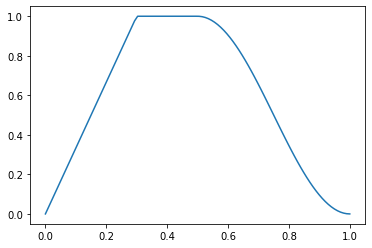source

### combined_cos

`` combined_cos (pct, start, middle, end)``

Return a scheduler with cosine annealing from `start``middle` & `middle``end`

This is a useful helper function for the 1cycle policy. `pct` is used for the `start` to `middle` part, `1-pct` for the `middle` to `end`. Handles floats or collection of floats. For example:

``````f = combined_cos(0.25,0.5,1.,0.)
plt.plot(p, [f(o) for o in p]);``````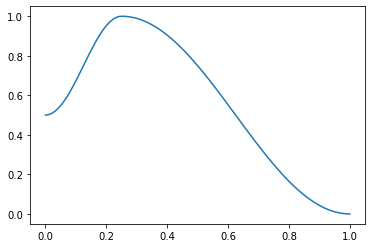source

### ParamScheduler

`` ParamScheduler (scheds)``

Schedule hyper-parameters according to `scheds`

`scheds` is a dictionary with one key for each hyper-parameter you want to schedule, with either a scheduler or a list of schedulers as values (in the second case, the list must have the same length as the the number of parameters groups of the optimizer).

``````learn = synth_learner()
sched = {'lr': SchedLin(1e-3, 1e-2)}
learn.fit(1, cbs=ParamScheduler(sched))
n = len(learn.dls.train)
test_close(learn.recorder.hps['lr'], [1e-3 + (1e-2-1e-3) * i/n for i in range(n)])``````
epoch train_loss valid_loss time
0 11.929138 4.039281 00:00

source

### ParamScheduler.before_fit

`` ParamScheduler.before_fit ()``

Initialize container for hyper-parameters

source

### ParamScheduler.before_batch

`` ParamScheduler.before_batch ()``

Set the proper hyper-parameters in the optimizer

source

### ParamScheduler.after_batch

`` ParamScheduler.after_batch ()``

Record hyper-parameters of this batch

source

### ParamScheduler.after_fit

`` ParamScheduler.after_fit ()``

Save the hyper-parameters in the recorder if there is one

source

### Learner.fit_one_cycle

`````` Learner.fit_one_cycle (n_epoch, lr_max=None, div=25.0,
div_final=100000.0, pct_start=0.25, wd=None,
moms=None, cbs=None, reset_opt=False,
start_epoch=0)``````

Fit `self.model` for `n_epoch` using the 1cycle policy.

The 1cycle policy was introduced by Leslie N. Smith et al. in Super-Convergence: Very Fast Training of Neural Networks Using Large Learning Rates. It schedules the learning rate with a cosine annealing from `lr_max/div` to `lr_max` then `lr_max/div_final` (pass an array to `lr_max` if you want to use differential learning rates) and the momentum with cosine annealing according to the values in `moms`. The first phase takes `pct_start` of the training. You can optionally pass additional `cbs` and `reset_opt`.

``````#Integration test: training a few epochs should make the model better
learn = synth_learner(lr=1e-2)
xb,yb = learn.dls.one_batch()
init_loss = learn.loss_func(learn.model(xb), yb)
learn.fit_one_cycle(2)
xb,yb = learn.dls.one_batch()
final_loss = learn.loss_func(learn.model(xb), yb)
assert final_loss < init_loss``````
epoch train_loss valid_loss time
0 19.444899 6.755066 00:00
1 9.919473 1.044571 00:00
``````#Scheduler test
lrs,moms = learn.recorder.hps['lr'],learn.recorder.hps['mom']
test_close(lrs,  [combined_cos(0.25,1e-2/25,1e-2,1e-7)(i/20) for i in range(20)])
test_close(moms, [combined_cos(0.25,0.95,0.85,0.95)(i/20) for i in range(20)])``````

source

### Recorder.plot_sched

`` Recorder.plot_sched (keys=None, figsize=None)``
``````learn = synth_learner()
learn.fit_one_cycle(2)``````
epoch train_loss valid_loss time
0 5.406837 5.305011 00:00
1 5.058437 4.899223 00:00
``learn.recorder.plot_sched()``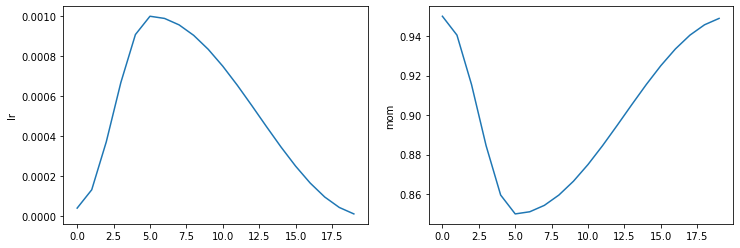source

### Learner.fit_flat_cos

`````` Learner.fit_flat_cos (n_epoch, lr=None, div_final=100000.0,
pct_start=0.75, wd=None, cbs=None, reset_opt=False,
start_epoch=0)``````

Fit `self.model` for `n_epoch` at flat `lr` before a cosine annealing.

``````learn = synth_learner()
learn.fit_flat_cos(2)``````
epoch train_loss valid_loss time
0 10.588930 7.106113 00:00
1 8.943380 5.016665 00:00
``learn.recorder.plot_sched()``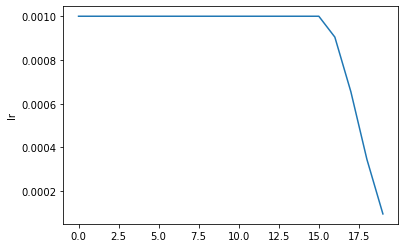source

### Learner.fit_sgdr

`````` Learner.fit_sgdr (n_cycles, cycle_len, lr_max=None, cycle_mult=2,
cbs=None, reset_opt=False, wd=None, start_epoch=0)``````

Fit `self.model` for `n_cycles` of `cycle_len` using SGDR.

This schedule was introduced by Ilya Loshchilov et al. in SGDR: Stochastic Gradient Descent with Warm Restarts. It consists of `n_cycles` that are cosine annealings from `lr_max` (defaults to the `Learner` lr) to 0, with a length of `cycle_len * cycle_mult**i` for the `i`-th cycle (first one is `cycle_len`-long, then we multiply the length by `cycle_mult` at each epoch). You can optionally pass additional `cbs` and `reset_opt`.

``````learn = synth_learner()
with learn.no_logging(): learn.fit_sgdr(3, 1)
test_eq(learn.n_epoch, 7)
iters = [k * len(learn.dls.train) for k in [0,1,3,7]]
for i in range(3):
n = iters[i+1]-iters[i]
#The start of a cycle can be mixed with the 0 of the previous cycle with rounding errors, so we test at +1
test_close(learn.recorder.lrs[iters[i]+1:iters[i+1]], [SchedCos(learn.lr, 0)(k/n) for k in range(1,n)])

learn.recorder.plot_sched()``````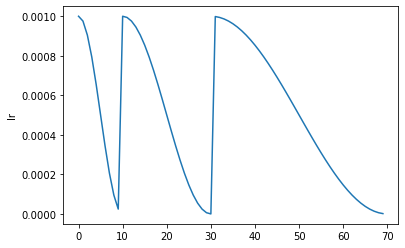source

### Learner.fine_tune

`````` Learner.fine_tune (epochs, base_lr=0.002, freeze_epochs=1, lr_mult=100,
pct_start=0.3, div=5.0, lr_max=None,
div_final=100000.0, wd=None, moms=None, cbs=None,
reset_opt=False, start_epoch=0)``````

Fine tune with `Learner.freeze` for `freeze_epochs`, then with `Learner.unfreeze` for `epochs`, using discriminative LR.

``learn.fine_tune(1)``
epoch train_loss valid_loss time
0 2.428970 1.740237 00:00
epoch train_loss valid_loss time
0 2.019952 1.616970 00:00

## Resume training from checkpoint

To enable resuming from checkpoint make sure to save model and optimizer state. This can be done using SaveModelCallback setting `(with_opt=True)`. If training is interrupted define `learn` using the same parameters as before, load model from checkpoint and pass `start_epoch` to `fit` call. The training will be resumed from `start_epoch` with properly scheduled `lr`.

``````with tempfile.TemporaryDirectory() as d:
learn1 = synth_learner(path=d, cbs=SaveModelCallback(with_opt=True, fname="ckpt"))
learn1.fit_one_cycle(5, cbs=InterruptCallback(2))

learn2 = synth_learner(path=d)
learn2.fit_one_cycle(5, start_epoch=2)

fig, axs = plt.subplots(1,2, sharey=True)
axs.plot(learn1.recorder.lrs)
axs.plot(learn2.recorder.lrs)``````
epoch train_loss valid_loss time
0 18.930223 14.100439 00:00
1 17.092665 10.603369 00:00
``````Better model found at epoch 0 with valid_loss value: 14.100439071655273.
Better model found at epoch 1 with valid_loss value: 10.603368759155273.``````
epoch train_loss valid_loss time
0 00:00
1 00:00
2 11.456764 10.057186 00:00
3 10.287196 8.694046 00:00
4 9.585465 8.422710 00:00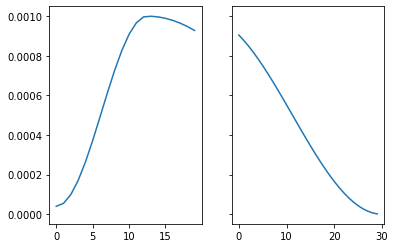source

### LRFinder

`` LRFinder (start_lr=1e-07, end_lr=10, num_it=100, stop_div=True)``

Training with exponentially growing learning rate

``from fastai.vision.all import *``
``````set_seed(99, True)
path = untar_data(URLs.PETS)/'images'

image_files = get_image_files(path)
if sys.platform == "win32" and IN_NOTEBOOK:
image_files = random.choices(image_files, k=int(len(image_files)/8))
print("Randomly select 1/8 files in NOTEBOOK on Windows to save time")

# pickle can't serializer lamda function.
def _label_func(x):
return x.isupper()

path, image_files, valid_pct=0.2,
label_func=_label_func, item_tfms=Resize(224))

learn = vision_learner(dls, resnet18)
learn.fit(1)
epoch train_loss valid_loss time
0 0.086690 0.016682 00:33
``````tensor([-5.8191e-04, -2.2443e-03,  0.0000e+00, -1.2517e-03,  0.0000e+00,
-1.4744e-03, -3.6433e-04,  0.0000e+00,  9.3745e-03,  0.0000e+00,
5.1993e-03, -1.5093e-02, -4.0410e-03,  0.0000e+00,  7.1963e-03,
-6.6033e-03, -3.3354e-03, -2.9191e-03, -1.5054e-03, -1.3179e-03,
8.7333e-03, -1.1155e-02, -9.6656e-04,  1.6653e-02,  9.5839e-04,
8.4995e-03, -2.8187e-02,  3.1579e-03, -9.3051e-04, -2.3887e-03,
-7.3557e-04, -1.4501e-02, -6.2110e-03,  1.9949e-03, -7.0233e-03,
1.2792e-02,  0.0000e+00,  1.0687e-03,  0.0000e+00, -4.2413e-04,
2.9628e-03,  7.2686e-03, -9.7241e-03, -4.9941e-04,  1.7408e-02,
-9.2441e-03, -9.7731e-03, -9.9393e-03,  0.0000e+00, -2.1448e-03,
2.7660e-03, -3.1110e-03,  5.9454e-05, -1.4412e-03, -6.1454e-04,
-1.6537e-03,  1.7001e-02,  1.4041e-02, -6.2878e-03,  2.0800e-02,
-1.2900e-02, -1.2626e-02, -2.6591e-03,  3.9685e-03], device='cuda:0')``````
``````with tempfile.TemporaryDirectory() as d:
learn = synth_learner(path=Path(d))
init_a,init_b = learn.model.a,learn.model.b
with learn.no_logging(): learn.fit(20, cbs=LRFinder(num_it=100))
assert len(learn.recorder.lrs) <= 100
test_eq(len(learn.recorder.lrs), len(learn.recorder.losses))
#Check stop if diverge
if len(learn.recorder.lrs) < 100: assert learn.recorder.losses[-1] > 4 * min(learn.recorder.losses)
#Test schedule
test_eq(learn.recorder.lrs, [SchedExp(1e-7, 10)(i/100) for i in range_of(learn.recorder.lrs)])
#No validation data
test_eq([len(v) for v in learn.recorder.values], [1 for _ in range_of(learn.recorder.values)])
test_eq(learn.model.a, init_a)
test_eq(learn.model.b, init_b)
test_eq(learn.opt.state_dict()['state'], [{}, {}])``````

source

### LRFinder.before_fit

`` LRFinder.before_fit ()``

Initialize container for hyper-parameters and save the model

source

### LRFinder.before_batch

`` LRFinder.before_batch ()``

Set the proper hyper-parameters in the optimizer

source

### LRFinder.after_batch

`` LRFinder.after_batch ()``

Record hyper-parameters of this batch and potentially stop training

source

### LRFinder.before_validate

`` LRFinder.before_validate ()``

Skip the validation part of training

### Suggestion Methods

There are a few methodologies for suggesting a learning rate automatically and these as we will see can further be passed into `lr_find`. Currently four methods are supported, however to write your own it should look like a function that can accept `LRFinder`’s returned `lrs`, `losses`, as well as the `num_it`. Your function should return an `x,y` coordinate that can be plotted, such as below:

``````def myfunc(lrs:list, losses:list, num_it:int) -> tuple(float, tuple(float,int)):
...
return suggestion, (suggestion,loss_idx)``````

If there are any more parameters to be passed in, you should pass in your `func` as a partial and specify them yourself, such as:

``````def myfunc(lrs:list, losses:list, num_it:int, pct_reduction:float) -> tuple(float, tuple(float,int)):
...
return suggestion, (suggestion,loss_idx)``````
``f = partial(myfunc, pct_reduction=.2)``

source

### valley

`` valley (lrs:list, losses:list, num_it:int)``

Suggests a learning rate from the longest valley and returns its index

The `valley` algorithm was developed by ESRI and takes the steepest slope roughly 2/3 through the longest valley in the LR plot, and is also the default for `Learner.lr_find`

source

### slide

`````` slide (lrs:list, losses:list, num_it:int, lr_diff:int=15,

Suggests a learning rate following an interval slide rule and returns its index

The `slide` rule is an algorithm developed by Andrew Chang out of Novetta, and is detailed here.

source

### minimum

`` minimum (lrs:list, losses:list, num_it:int)``

Suggests a learning rate one-tenth the minumum before divergance and returns its index

source

### steep

`` steep (lrs:list, losses:list, num_it:int)``

Suggests a learning rate when the slope is the steepest and returns its index

source

### Recorder.plot_lr_find

`````` Recorder.plot_lr_find (skip_end=5, return_fig=True, suggestions=None,
nms=None, **kwargs)``````

Plot the result of an LR Finder test (won’t work if you didn’t do `learn.lr_find()` before)

source

### Learner.lr_find

`````` Learner.lr_find (start_lr=1e-07, end_lr=10, num_it=100, stop_div=True,
show_plot=True, suggest_funcs=<function valley>)``````

Launch a mock training to find a good learning rate and return suggestions based on `suggest_funcs` as a named tuple

First introduced by Leslie N. Smith in Cyclical Learning Rates for Training Neural Networks, the LR Finder trains the model with exponentially growing learning rates from `start_lr` to `end_lr` for `num_it` and stops in case of divergence (unless `stop_div=False`) then plots the losses vs the learning rates with a log scale.

A variety of learning rate suggestion algorithms can be passed into the function, by default we use the `valley` paradigm.

``````with tempfile.TemporaryDirectory() as d:
learn = synth_learner(path=Path(d))
weights_pre_lr_find = L(learn.model.parameters())
lr_min, lr_steep, lr_valley, lr_slide = learn.lr_find(suggest_funcs=(minimum, steep, valley, slide))
weights_post_lr_find = L(learn.model.parameters())
test_eq(weights_pre_lr_find, weights_post_lr_find)
print(f"Minimum/10:\t{lr_min:.2e}\nSteepest point:\t{lr_steep:.2e}\nLongest valley:\t{lr_valley:.2e}\nSlide interval:\t{lr_slide:.2e}")``````
``````Minimum/10: 1.58e-01
Steepest point: 9.12e-03
Longest valley: 1.58e-02
Slide interval: 8.32e-02``````